## A box with mass 15.0 kg moves on a ramp that is inclined at an angle of 55.0∘ above the horizontal. The coefficient of kinetic friction betw

Question

A box with mass 15.0 kg moves on a ramp that is inclined at an angle of 55.0∘ above the horizontal. The coefficient of kinetic friction between the box and the ramp surface is μk = 0.300.
a. Calculate the magnitude of the acceleration of the box if you push on the box with a constant force 190.0 N that is parallel to the ramp surface and directed down the ramp, moving the box down the ramp.
b. Calculate the magnitude of the acceleration of the box if you push on the box with a constant force 190.0 N that is parallel to the ramp surface and directed up the ramp, moving the box up the ramp.

in progress 0
4 months 2021-09-05T07:18:36+00:00 1 Answers 5 views 0

a) a = 19.0 m/s²

b) a = 2.9 m/s²

Explanation:

a) We draw the free body diagram of the box. There are 4 forces: the normal force N, the weight mg, the constant force F and the kinetic frictional force μ_kN. We can take the coordinate system which is rotated 55° from the horizontal, to ease the calculations. So, we write the equations of motion in each axis: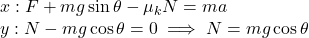Substituting the expression for N in the first equation, we have: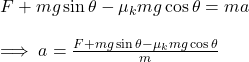If we plug in the given values, we have: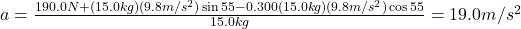Since we chose the right-downward direction as positive, the positive sign in this case means that the box is accelerated downwards above the ramp.

b) In this case, the constant force F and the kinetic frictional force μ_kN point to the opposite side. In other words, we can just only change the sign of this two forces in the equations of part (a) and obtain: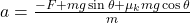Plugging in the given values: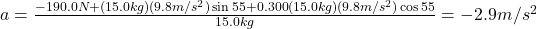Since we chose the right-downward direction as positive, the negative sign in this case means that the box is accelerated upwards above the ramp.

This means that the magnitude of the acceleration in this case is 2.9m/s².